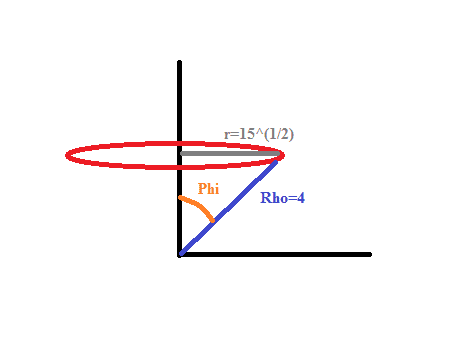# Triple integral

Hi, I have to compute de volume for the region:

$$\{(x,y,z):x^2+y^2+z^2\leq{16};z\geq{1} \}$$

I've tried to do by two different parametrizations, in spherics and cylindrical coordinates

$$3 \displaystyle\int_{0}^{\sqrt[ ]{15}}\int_{0}^{2\pi}\int_{1}^{\sqrt[ ]{16-r^2}}dz d \theta dr\approx{162.26}$$

For spherics I've considered $$4\sin \phi=\sqrt[ ]{15}\Rightarrow{\phi=Arcsen\left( \displaystyle\frac{\sqrt[ ]{15}}{4} \right)}=u$$

$$3 \displaystyle\int_{0}^{u}\int_{0}^{2\pi}\int_{\cos \phi}^{4}d\rho d \theta d\phi\approx{81.13 }$$

I've used wolfram to make the calculus, and it gives that for cylindrical coord. I'm having twice the volume that I have for spherical coord. I don't know where I'm committing the mistake. Bye, thanks for helping :)

Note: I'm multiplying by 3 because I was going to use for Gauss theorem and is the divergence for the vector field given by the problem

wheres your Jacobian for spherical and cylindrical coordinates.

Thanks :P I forgot about that, I thought I wasn't making any change of variables, but now I see. It's because I've been doing some surface integrals and the jacobian doesn't appear on those kind of integrals, I'm not sure why is that, but I think that role is played by the normal vector, which is pretty much like the jacobian.

Last edited:
Well, I added the jacobians, but still giving different, I don't know why. The difference now is more sutil: In cylindrical coord. gives (clic here)

In spherical coord gives

I tried to proceed with cylindrical, but the exercise gets more complicated, so I want to be sure that what I'm doing with spherical is ok, so I can proceed that way, but still giving different answers.

Last edited:
I think you bound for phi in spherical should be $\phi=arctan(\sqrt{15})$
and not your arcsin that you have.

why is that? I've reasoned the bound this way:Then $$4 \sin \phi=\sqrt[]{15}$$

#### Attachments

vela
Staff Emeritus
Homework Helper
That gives the same limits because you have, equivalently,
\begin{align*}
\cos \phi &= \frac{1}{4} \\
\sin \phi &= \sqrt{1-\left(\frac{1}{4}\right)^2} = \frac{\sqrt{15}}{4} \\
\tan \phi &= \frac{\sin\phi}{\cos\phi} = \sqrt{15}
\end{align*}
I think the lower bound of $\rho$ is wrong.

Oh, yes, it says cos phi, but I used secant in wolfram. I'll correct the typo, thanks vela.

I can´t edit now the first post, but this is the integration I've computed with wolfram:

$$3 \displaystyle\int_{0}^{u}\int_{0}^{2\pi}\int_{\sec \phi}^{4}\rho^2 \sin \phi d\rho d \theta d\phi=81\pi$$

And for cylindrical:

$$3 \displaystyle\int_{0}^{\sqrt[ ]{15}}\int_{0}^{2\pi}\int_{1}^{\sqrt[ ]{16-r^2}}r dz d \theta dr\approx{80\pi}$$

their equivalent i just did it with tangent. Yours is fine . It looks like your bounds are right but i did both integral and got 80 and 100 . so i got different answers but maybe i made a mistake.

vela
Staff Emeritus
Homework Helper
I got $81\pi$ for both using Mathematica.

Thanks vela, perhaps it's only a bug in wolfram alpha :)

$$3 \displaystyle\int_{0}^{\sqrt[ ]{15}}\int_{0}^{2\pi}\int_{1}^{\sqrt[ ]{16-r^2}}r dz d \theta dr=80\pi$$

This is actually right, I just corroborated it doing it by hand:

$$3 \displaystyle\int_{0}^{\sqrt[ ]{15}}\int_{0}^{2\pi}\int_{1}^{\sqrt[ ]{16-r^2}}r dz d \theta dr=3 \displaystyle\int_{0}^{\sqrt[ ]{15}}\int_{0}^{2\pi}(r\sqrt[ ]{16-r^2}-r)d \theta dr$$
Using:
$$u=16-r^2\rightarrow du=-2r$$
$$3 \displaystyle\int_{0}^{\sqrt[ ]{15}}\int_{0}^{2\pi}(r\sqrt[ ]{16-r^2}-r)d \theta dr=-3\pi\displaystyle\int_{16}^{0}\sqrt[ ]{u}du-6\pi \left( \frac{r^2}{2} \right ) _0^4=2\pi 16^{3/2}-48\pi=80\pi$$

vela
Staff Emeritus
Homework Helper
You got the limits for u wrong and plugged in the wrong limits for the other term.

Uups you're right. That was the mistake, thank you vela :) I made a big mess with this involving spherical and cylindrical coordinates :P but the thing is that for the volume it's easier to compute it using cylindrical coordinates (unless I think so). But for the surface integrals spheric coordinates seems to be better (gives simpler expressions).

I wanted to corroborate the Gauss theorem for that region, the problem gives a vector field. I have some doubts about that too, I should make another topic for that, right?

vela
Staff Emeritus